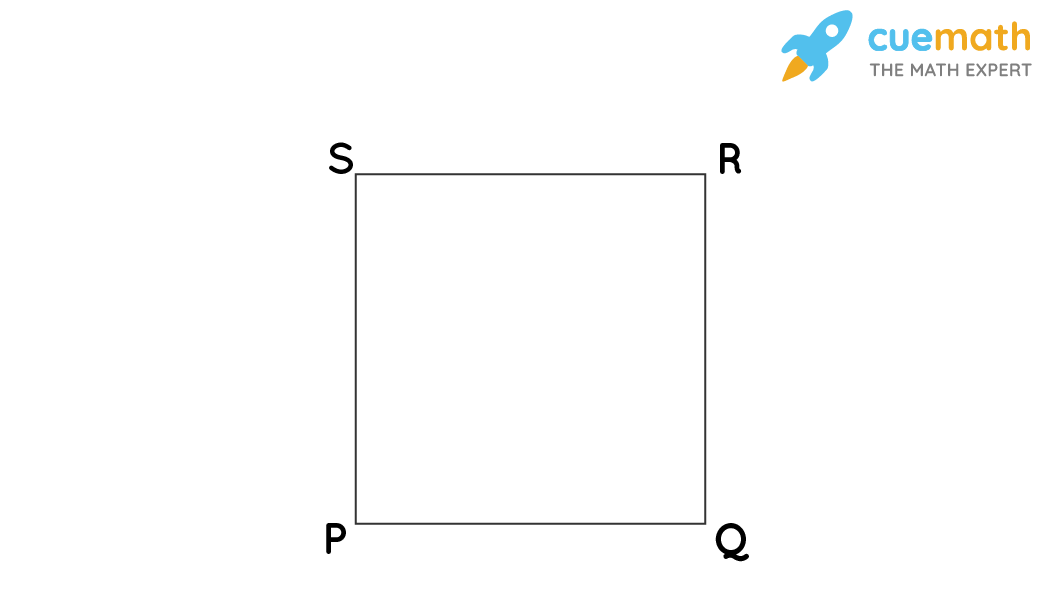# Given Ab Explain How to Construct a Square With Sides of Length Ab

## Question: Given AB explain how to construct a square with sides of length AB?

A square is a closed two-dimensional figure with four sides and four corners. The length of all four sides is equal and parallel to each other.

## Answer: The steps to construct a square with sides of length AB are given below.

Here is a step-by-step process for you to construct a square of length AB using a ruler and a compass.

## Explanation:

These are the following steps to construct a square of Length PQ.

• Step 1: Draw a line segment PQ of any dimension.
• Step 2: Extend the same line PQ. Use a compass to draw two arcs on each side of Q and name the points as U and V.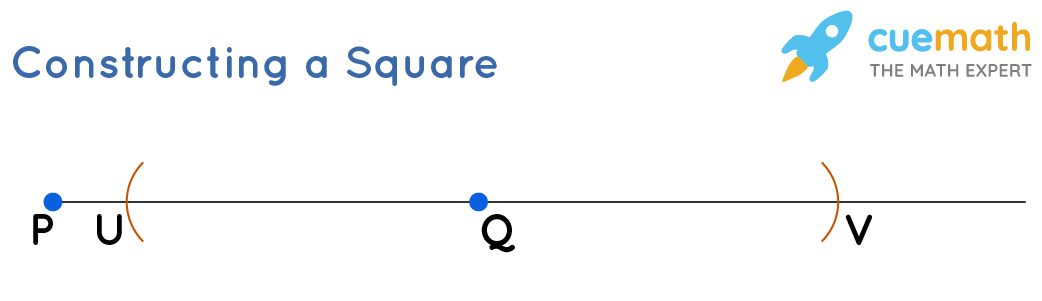• Step 3: Using the compass from points U and V, draw arcs above point Q. The point where the arcs meet is named W.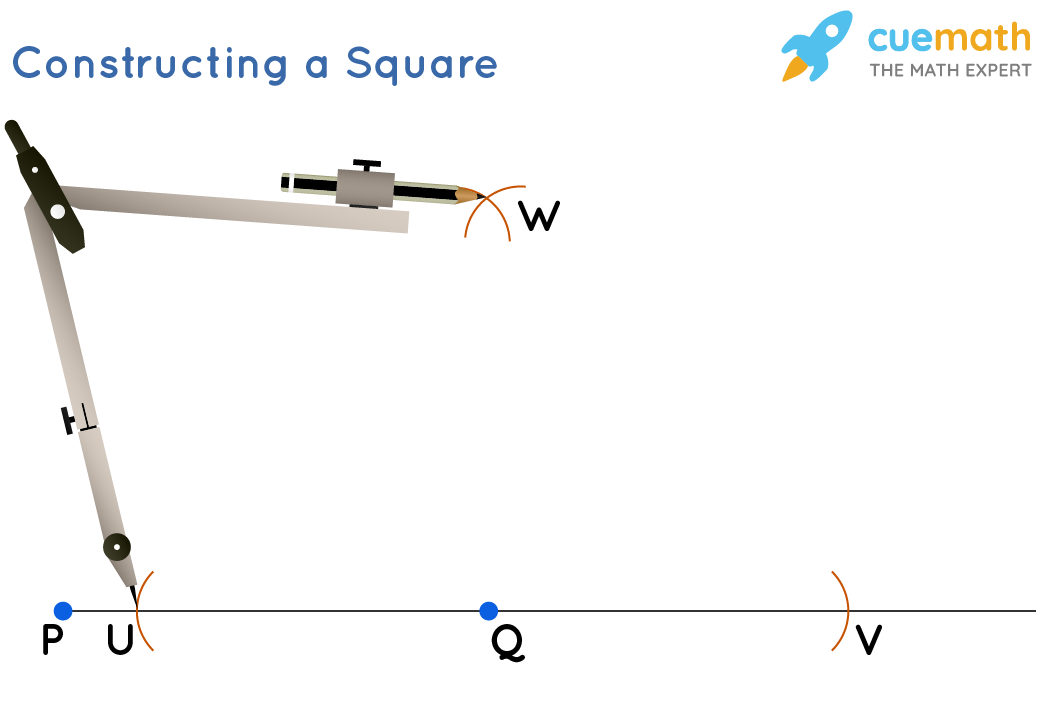• Step 4: Now, draw a line from Q to W.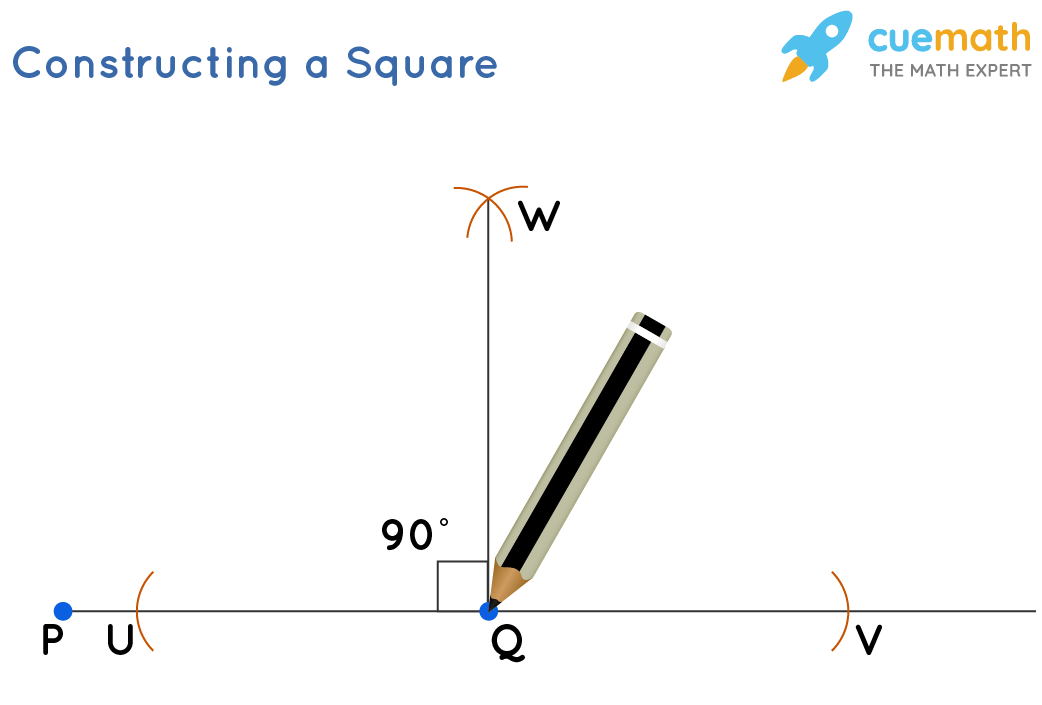• Step 5: Set the compass to any radius. Draw an arc from point P above the same point. Now move the compass with the same radius to point Q and draw an arc across QW and named it as R. This is the vertex of the square.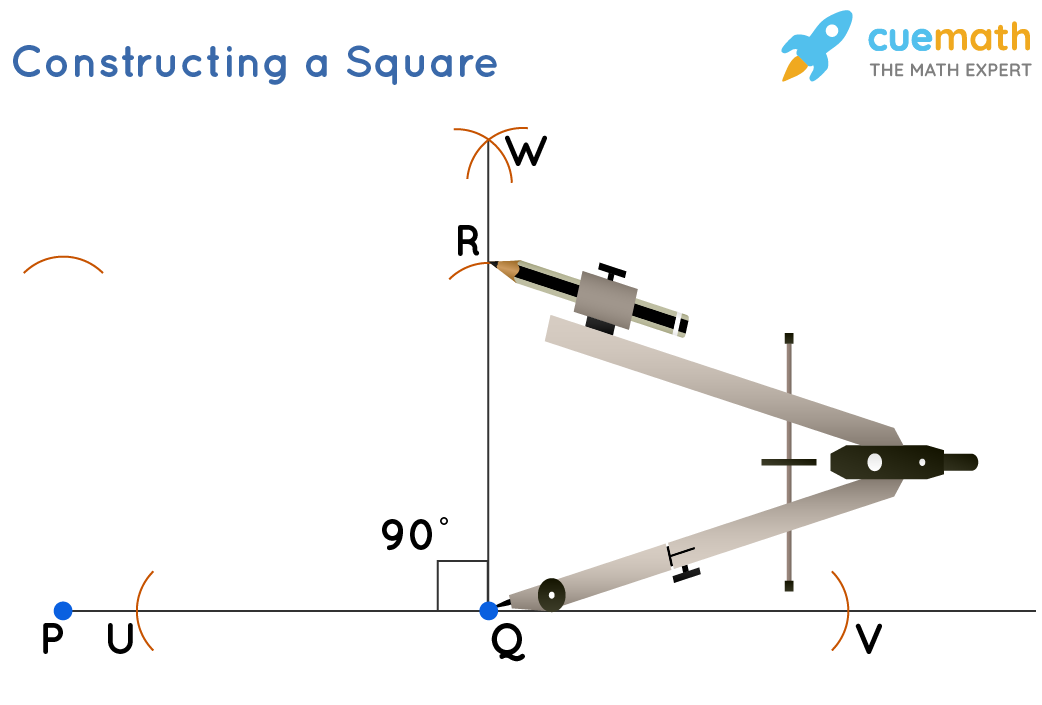• Step 6: Using the compass with the same radius, draw an arc from point R on the previously drawn arc above point P. The point of intersection is named S.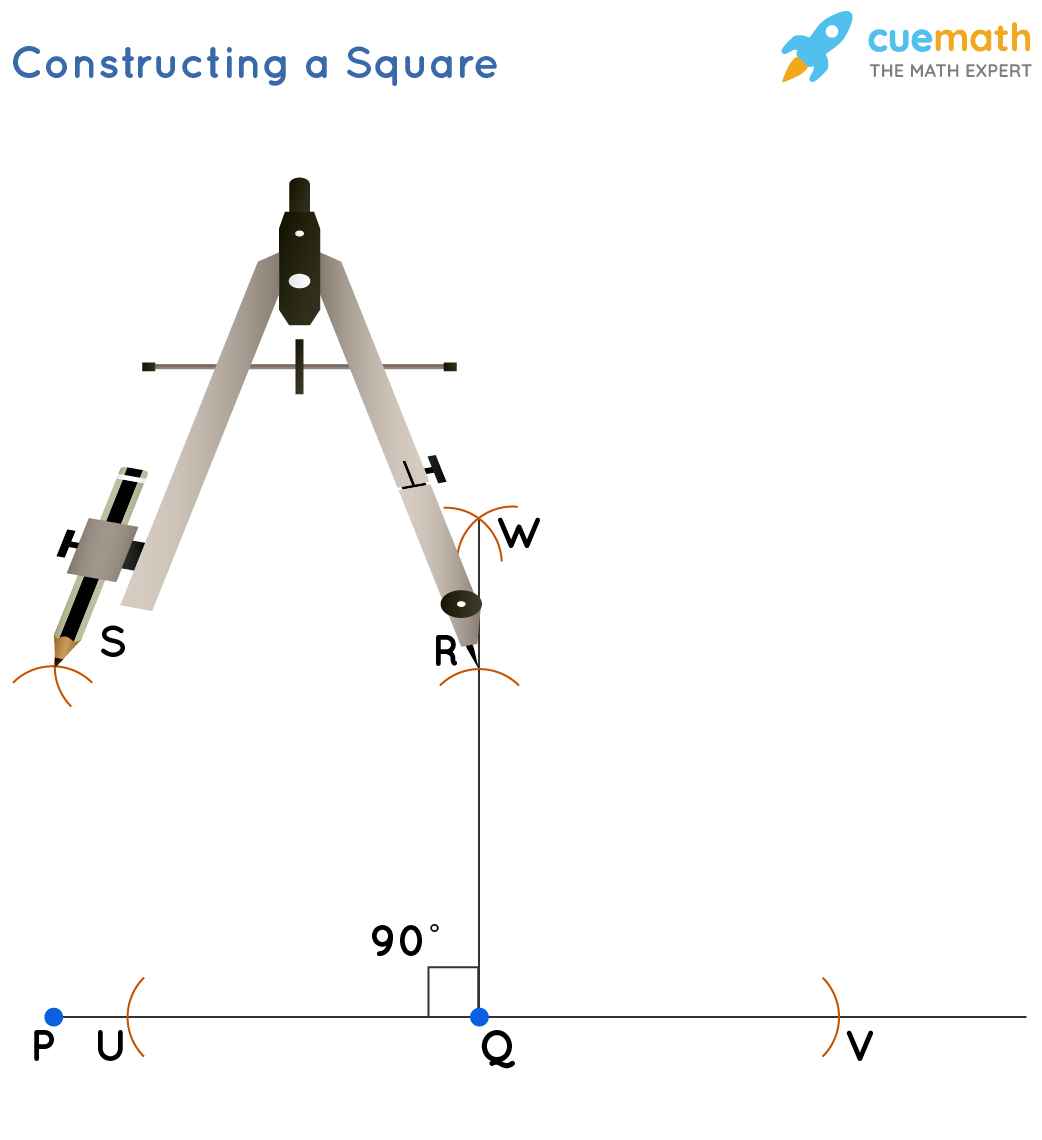• Step 7: Join the points R and S as well as P and S to get the square PQRS.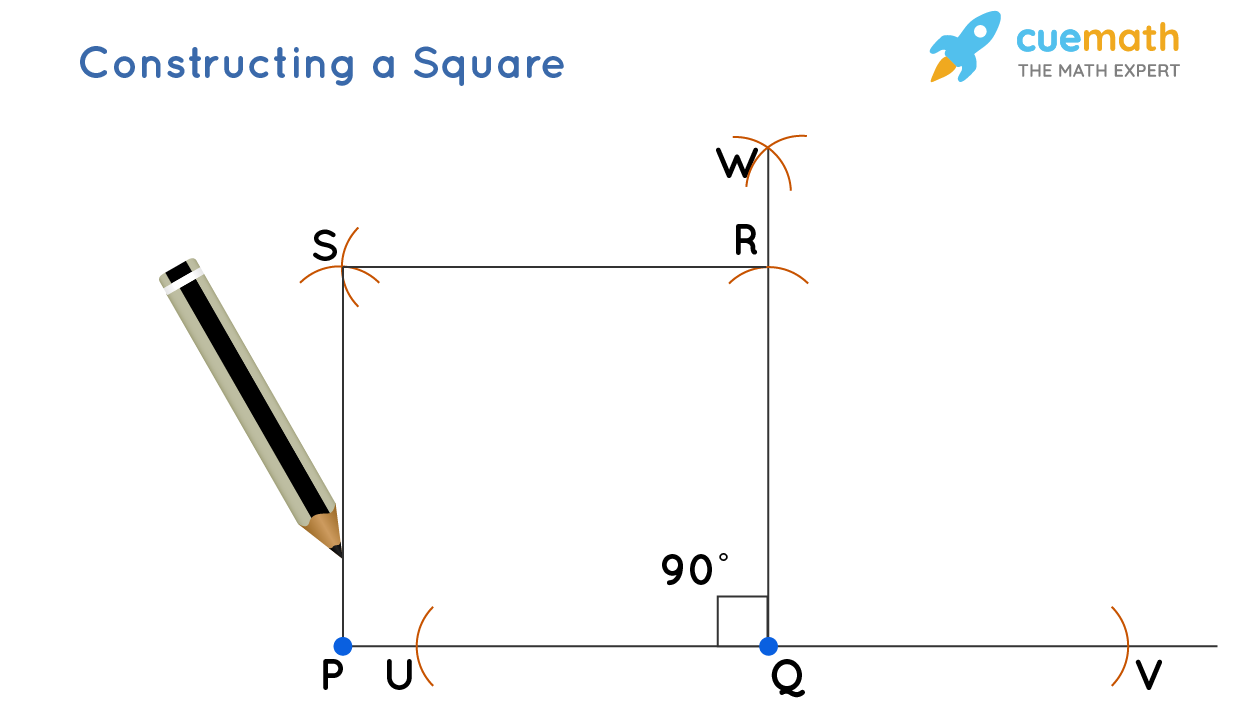• Step 8: Erase all lines and arcs except the ones made in the last two steps. The final figure is that of a square.